ISEE Middle Level Math : How to find the square root

Example Questions

Example Question #251 : How To Find The Square Root

What is the value of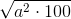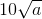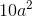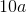Explanation:

In order to solve for, the square root must be taken of 100 and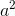, which results in 10a, the correct answer.

Example Question #252 : How To Find The Square Root

Solve: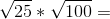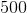Explanation:

First find the two square roots: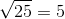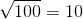Then multiply: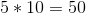Answer:Example Question #253 : How To Find The Square Root

Solve: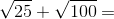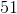Explanation:

Find the square roots: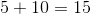Answer:Example Question #254 : How To Find The Square Root

Solve: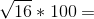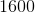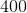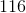Explanation:

First find the square root: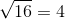Then multiply by 100: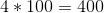Answer:Example Question #255 : How To Find The Square Root

Solve: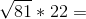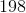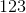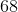Explanation:

First find the square root: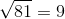Then perform the multiplication: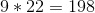Answer:Example Question #256 : How To Find The Square Root

Solve: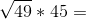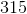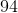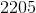Explanation:

According to the order of operations, we should first simplify the radical, then multiply: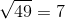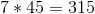Answer:Example Question #257 : How To Find The Square Root

Solve: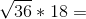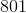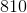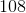Explanation:

We should always simplify when possible. Let's first simplify the square root: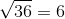Now all that's left is to find the product: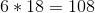Answer:Example Question #258 : How To Find The Square Root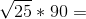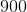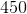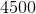Explanation:

First take the square root:Then multiply: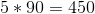Answer:Example Question #259 : How To Find The Square Root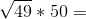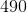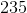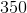Explanation:

First find the square root, then the product: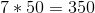Answer:Example Question #260 : How To Find The Square Root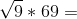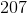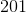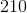Explanation:

Take the square root: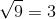Multiply: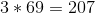Answer: